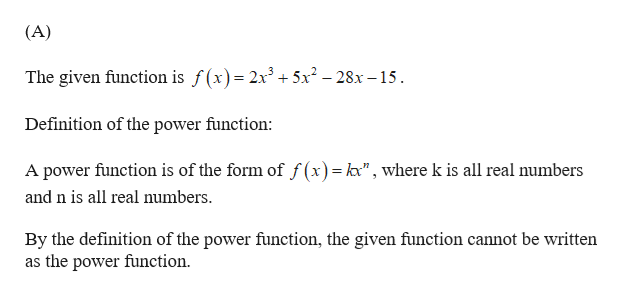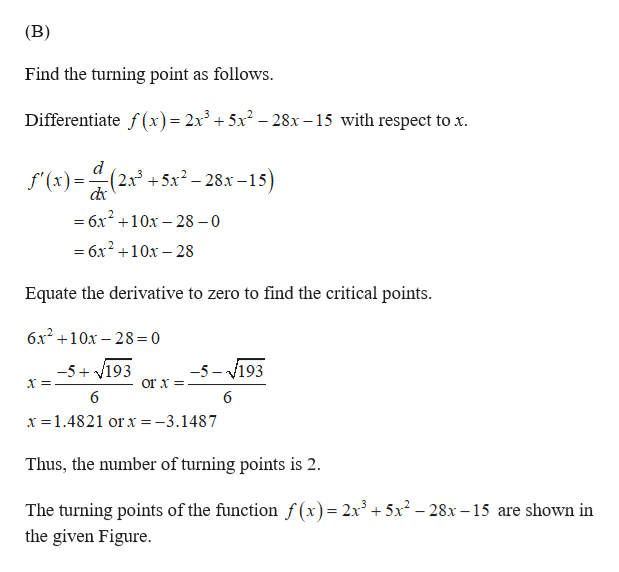# g(x)=2x^3+5x^2-28x-15A)find power functionB) homany turning points can this graph have

Question
24 views

g(x)=2x^3+5x^2-28x-15

A)find power function

B) homany turning points can this graph have

check_circle

Step 1help_outlineImage Transcriptionclose(A) The given function is f(x)= 2x3 + 5x2-28x -15 Definition of the power function: A power function is of the form of f(x) = k", where k is all real numbers and n is all real numbers By the definition of the power function, the given function cannot be written as the power function fullscreen
Step 2help_outlineImage Transcriptionclose(B) Find the turning point as follows Differentiate f(x) = 2x3 + 5x2 - 28x -15 with respect to x (2x3 +5x2-28x-15) f'(x) =6x 10x-28 0 =6x2 10x-28 Equate the derivative to zero to find the critical points. 6x210x 28= 0 -5-193 -5 193 or x 6 6 x 1.4821 or x =-3.1487 Thus, the number of turning points is 2 The turning points of the function f (x)= 2x3 +5x2 -28x -15 are shown in the given Figure. fullscreen

### Want to see the full answer?

See Solution

#### Want to see this answer and more?

Solutions are written by subject experts who are available 24/7. Questions are typically answered within 1 hour.*

See Solution
*Response times may vary by subject and question.
Tagged in

### Calculus